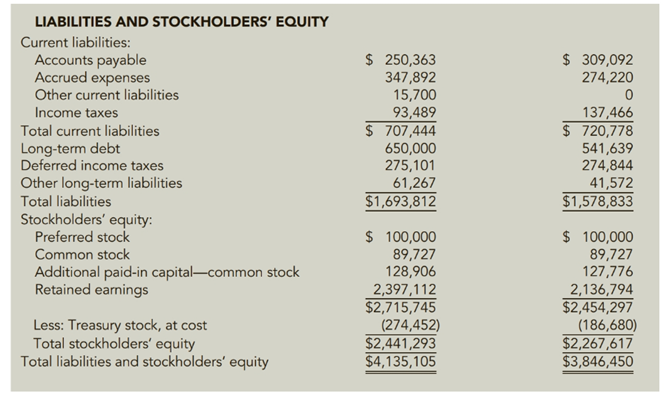Chapter 12, Problem 76E### Cornerstones of Financial Accounti...

4th Edition
Jay Rich + 1 other
ISBN: 9781337690881

#### Solutions

Chapter
Section### Cornerstones of Financial Accounti...

4th Edition
Jay Rich + 1 other
ISBN: 9781337690881
Textbook Problem
5 views

# Preparation of Common Size Statements for Vertical AnalysisFinancial statements for Steele Inc. follow.Required:1. Prepare common size income statements and balance sheets for Steele to be used in vertical analysis (in percentage terms, rounded to two decimal places). You do not need to include the actual dollar amounts shown above.2. CONCEPTUAL CONNECTION Indicate whether gross margin grew as much as sales between 2017 and 2018 and between 2018 and 2019, and, if so, why it grew.3. CONCEPTUAL CONNECTION Indicate whether the relative proportion of Steele’s assets changed between 2018 and 2019, and. if so, explain the change.4. CONCEPTUAL CONNECTION Indicate whether the relative proportion of Steele’s liabilities and equity changed between 2018 and 2019, and. if so. explain the change.5. CONCEPTUAL CONNECTION Explain how Steele appears to have financed the 7.5% increase in assets that occurred between 2018 and 2019.

To determine

(a)

Introduction:

Under vertical analysis, each item of financial statement is shown as a percent of the highest amount available on the same statement.

To prepare:

Common size financial statements for vertical analysis.

Explanation

COMMON SIZE INCOME STATEMENT

 Particulars 2019 2018 2017 Net Sales $7,245,088$7,245,088×100 = 100% $6,944,296$6,944,296×100 = 100% $6,149,218$6,149,218×100 = 100% Cost of Goods Sold $5,286,253$7,245,088×100 = 72.96% $4,953,556$6,944,296×100 = 71.33% $4,355,675$6,149,218×100 = 70.83% Gross Margin $1,958,835$7,245,088×100 = 27.04 $1,990,740$6,944,296×100 = 28.67% $1,793,543$6,149,218×100 = 29.17% General and administrative expenses $1,259,896$7,245,088×100 = 17.39% $1,202,042$6,944,296×100 = 17.31% $1,080,843$6,149,218×100 = 17.58% Special and nonrecurring items $2,617$7,245,088×100 = 0.04% $0$6,944,296×100 = 0 $0$6,149,218×100 = 0 Operating Income $701,556$7,245,088×100 = 9.69% $788,698$6,944,296×100 = 11.36% $712,700$6,149,218×100 = 11.59% Interest expense $63,685$7,245,088×100 = 0.89% $62,398$6,944,296×100 = 0.90% $63,927$6,149,218×100 = 1.04% Other Income $7,308$7,245,088×100 = 0.10% $10,080$6,944,296×100 = 0.15% $11,529$6,149,218×100 = 0.19% Gain on Sale of Investments $0$7,245,088×100 = 0 $9,117$6,944,296×100 = 0.13% $0$6,149,218×100 = 0 Income for income taxes $645,179$7,245,088×100 = 8.91% $745,497$6,944,296×100 = 10.74% $660,302$6,149,218×100 = 10.74% Provision for income taxes $254,000$7,245,088×100 = 3.51% $290,000$6,944,296×100 = 4.18% $257,000$6,149,218×100 = 4.18% Net Income $391,179$7,245,088×100 = 5.40% $455,497$6,944,296×100 = 6.56% $403,302$6,149,218×100 = 6.56%

COMMON SIZE BALANCE SHEET

 ASSETS Dec 31, 2019 Dec 31, 2018 Current Assets: Cash and equivalents $320,558$4,135,105×100 = 7.75% $41,235$3,846,450×100 = 1.07% Accounts Receivable $1,056,911$4,135,105×100 = 25.56% $837,377$3,846,450×100 = 21.77% Inventories $733,700$4,135,105×100 = 17.74% $803,707$3,846,450×100 = 20.89% Other $109,456$4,135,105×100 = 2
To determine

(b)

Introduction:

When a company holds 50% or more of the common stock of another company then both the companies are treated as a single entity. In such a case, consolidated statements are prepared.

To compare:

The growth in gross margin and sales between year 2017 and 2018, 2018 and 2019.

To determine

(c)

Introduction:

When a company holds 50% or more of the common stock of another company then both the companies are treated as a single entity. In such a case, consolidated statements are prepared.

To compare:

Relative proportion of assets between 2018 and 2019.

To determine

(d)

Introduction:

When a company holds 50% or more of the common stock of another company then both the companies are treated as a single entity. In such a case, consolidated statements are prepared.

To compare:

Relative proportion of liabilities and equity between 2018 and 2019.

To determine

(d)

Introduction:

When a company holds 50% or more of the common stock of another company then both the companies are treated as a single entity. In such a case, consolidated statements are prepared.

The source of finance for the increased value of asset.

### Still sussing out bartleby?

Check out a sample textbook solution.

See a sample solution

#### The Solution to Your Study Problems

Bartleby provides explanations to thousands of textbook problems written by our experts, many with advanced degrees!

Get Started

#### Find more solutions based on key concepts